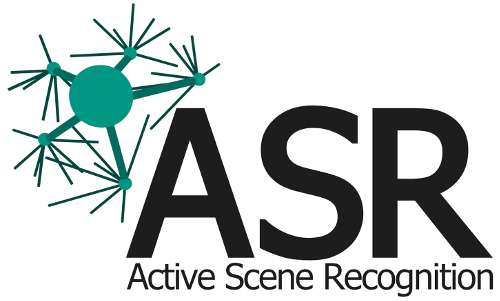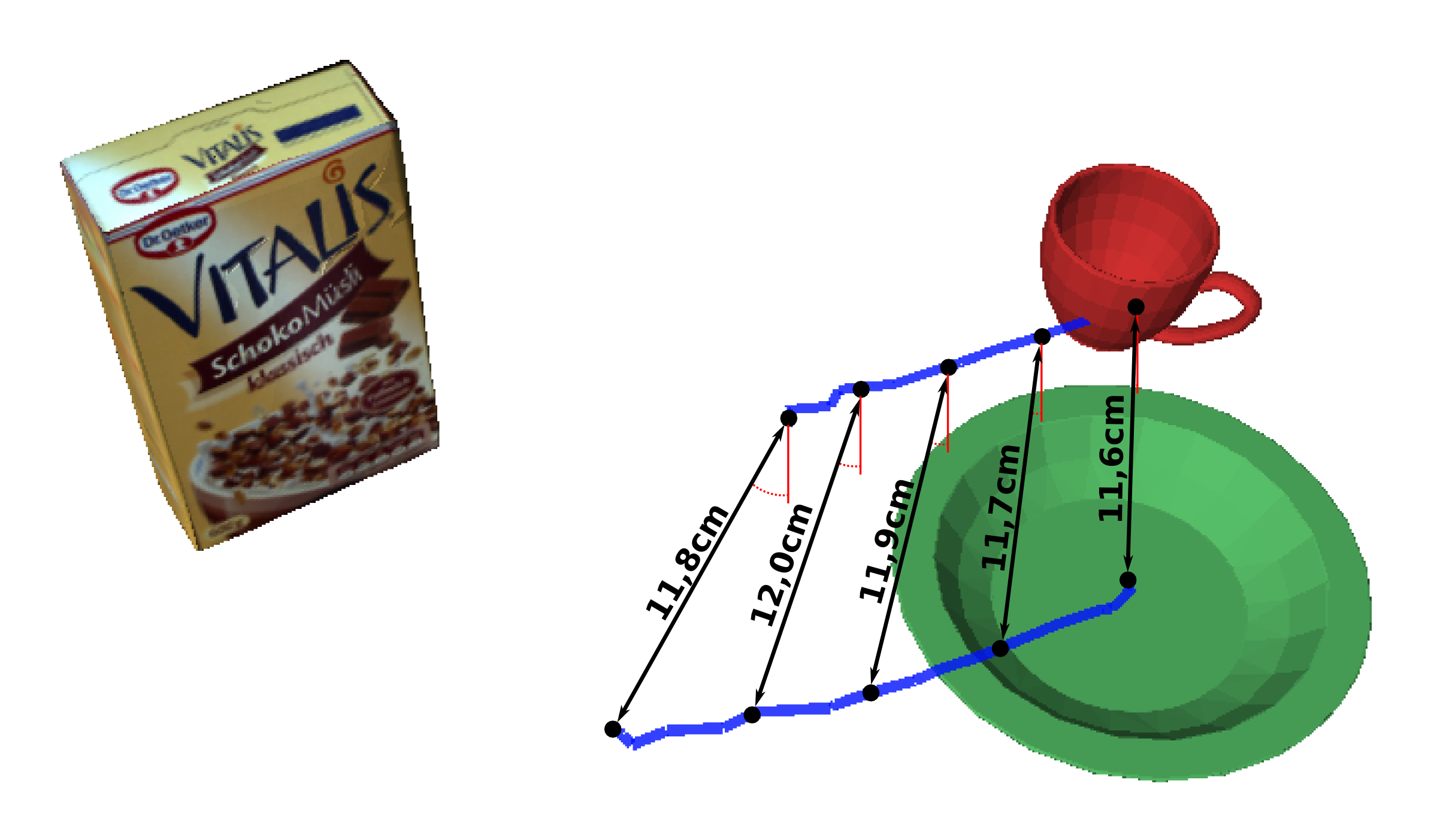[Documentation] [TitleIndex] [WordIndex]

# Package Summary

Generates the tree containing selected relations between scene objects from recorded trajectories. Used in PSM.

# Package Summary

Generates the tree containing selected relations between scene objects from recorded trajectories. Used in PSM.## Description

asr_relation_graph_generator, used by asr_psm, generates a tree or graph containing selected relations between the objects of a scene.

## Functionality

PSMTrainer

Library functions get called with ISM::ObjectSet messages representing observed object trajectories from which the relations between the objects are chosen. PSMTrainer uses agglomerative clustering to choose relevant relations based on a heuristic, attempting to represent the global neighbourhood of each object while simultaneously keeping the number of relations as low as possible, since more relations mean higher learning and inference time in asr_psm. The heuristic considers the parallelity of object trajectories. It outputs a SceneModel::TreeNode representing the root of the generated tree.The blue lines show the trajectories of the cup and the plate. The distance between them at each step is shown in black, the angles in red.

FullyMeshedTrainer

Generates a graph containing all possible object relations. Precise results, but long recognition runtime.

TopologyTreeTrainer

Generates a graph representing a given set of Relations.

TopologyCreator

The TopologyCreator is a helper used to generate different topologies, that is, relation sets, for instance the fully meshed or star-shaped ones. It can also generate a random one as well as neighbours of a given set.

## Usage

### Start system

Called from asr_psm, see there for how it is used in context.

## ROS Nodes

### Parameters

PSMTrainer

Set in asr_psm launch files:

• static_break_ratio: static breaks are misalignments in angle between the objects at different trajectory steps. If there are more static breaks than static_break_ratio (multiplied with the sample range), two trajectories are considered not parallel. Default: 1.01.

• together_ratio: if the two objects do not appear together in more than together_ratio of the trajectory steps, they are considered not parallel as well. Default: 0.9.

• max_angle_deviaton: the value in degrees above which an angle is considered a misalignment. Default: 45.

All are used by the heuristic to judge the parallelity of trajectories to in each step cluster the two best fits together.

The other trainers don't have specific parameters.

Use the parameter relation_tree_trainer_type to specify which trainer is to be used. tree is the PSMTrainer, fully_meshed the FullyMeshedTrainer. The TopologyTreeTrainer is used during combinatorial_optimization.

TopoloyCreator

The relevant parameters for the TopologyCreator are:

• remove_relations: whether to use removal of relations as operation in neighbour generation. Default: true.

• swap_relations: whether to use swapping of relations as operation in neighbour generation. Default: true.

• maximum_neighbour_count: maximum number of neighbours to select in each step. Default: true.

The third operation in neighbour creation is adding a relation, which cannot be dactivated.

## Tutorials

See asr_psm for use in context.

PSMTrainer

In Code:

In ProbabilisticSceneModel::OcmForegroundSceneLearner::learn():

```// generate trainer. Ratios and deviation from ros parameters:
SceneModel::PSMTrainer trainer(staticBreakRatio, togetherRation, maxAngleDeviation);

// which describes the observed scene object trajectories

// create tree:

...

// print tree. The 0 indicates the tree depth level.
trainer.getTree()->printTreeToConsole(0);

//learn a model with the tree as its base:
learner->learn(examplesList, trainer.getTree());```

The other trainers function similarly, but take other parameters in construction:

FullyMeshedTrainer: none

TopologyTreeTrainer:

`TopologyTreeTrainer(std::vector<boost::shared_ptr<Relation>> pRelations);`

Where pRelations are the relations representing the topology to be transformed into a graph, or rather, a tree containing reference nodes (hence the name).

TopologyCreator

Use one of the functions to get a Topology representing a relation set.

The central functions are:

`std::vector<boost::shared_ptr<Topology>> generateNeighbours(boost::shared_ptr<Topology> pFrom);`

Generates a set of neighbouring topologies from the given one using up to three operations depending on the parameters passed in constructor (see parameters).

`std::vector<boost::shared_ptr<Topology>> generateStarTopologies();`

Generates all possible star-shaped topologies containing all the object types passed in constructor.

`boost::shared_ptr<Topology> generateFullyMeshedTopology();`

Generates the fully meshed topology containing all the object types from constructor.

`boost::shared_ptr<Topology> generateRandomTopology();`

Generates a random connected topology containing the object types.

2021-01-16 12:32